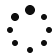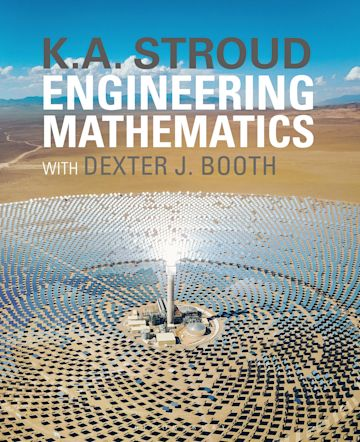## Build your students’ confidence and skills in Engineering Maths with Maths Engine.

### What is Maths Engine?

Maths Engine is a fusion of our bestselling textbook, Stroud's Engineering Mathematics and an innovative online assessment platform, offering endless practice options, instant feedback and an integrated eBook.

Questions map to practice equations in Engineering Mathematics, closely mirroring and reinforcing the supportive, step-by-step approach of the book, which has been trusted by lecturers and students for over 50 years.

Whether you’re looking for a resource to work alongside Engineering Mathematics, a self-study platform for your students to use for additional practice or are looking to direct your students’ learning via assignments and tests, Maths Engine will support students as they boost their knowledge and skills, allowing them to perform at their best.

### ▼ Topics covered

• Arithmetic
• Algebra
• Expressions and equations
• Graphs
• Linear equations
• Polynomial equations
• Binomials
• Partial fractions
• Triginometry
• Functions
• Trigonometric and exponential equations
• Differentiations
• Integration
• Complex numbers
• Hyperbolic functions
• Determinants
• Matrices
• Vectors
• Differentiation
• Differentiation applications
• Tangent, normal and curvature
• Sequences
• Curves and curve fitting
• Reduction formulas
• Polar coordinate systems
• Multiple integrals
• Introduction to Laplace transforms
• Data handling and statistics
• Probability